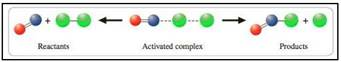# Sketch a potential-energy diagram for the decomposition of nitrous oxide. N 2 O ( g ) → N 2 ( g ) + O ( g ) The activation energy for the forward reaction is 251 kJ; the Δ H° is +167 kJ. What is the activation energy for the reverse reaction? Label your diagram appropriately.### General Chemistry - Standalone boo...

11th Edition
Steven D. Gammon + 7 others
Publisher: Cengage Learning
ISBN: 9781305580343### General Chemistry - Standalone boo...

11th Edition
Steven D. Gammon + 7 others
Publisher: Cengage Learning
ISBN: 9781305580343

#### Solutions

Chapter
Section
Chapter 13, Problem 13.76QP
Textbook Problem
461 views

## Sketch a potential-energy diagram for the decomposition of nitrous oxide. N 2 O ( g ) → N 2 ( g ) + O ( g ) The activation energy for the forward reaction is 251 kJ; the ΔH° is +167 kJ. What is the activation energy for the reverse reaction? Label your diagram appropriately.

Expert Solution
Interpretation Introduction

Interpretation:

The activation energy for the reverse reaction has to be calculated along with labeled diagram has to be given.

Concept Introduction:

Transition state theory deals about the collision of two molecules results in reaction that is done by an activated complex. An activated complex is group of unstable atoms, which breaks up to form products.Figure 1

### Explanation of Solution

To calculate the activation energy and give labeled sketch

The activation energy of forward reaction = +251 kJ

ΔH°=+167

### Want to see the full answer?

Check out a sample textbook solution.See solution

### Want to see this answer and more?

Bartleby provides explanations to thousands of textbook problems written by our experts, many with advanced degrees!

See solution